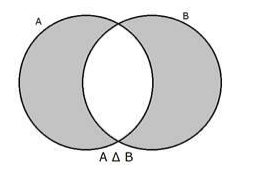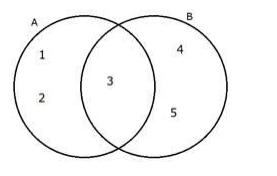# Find the symmetric difference A Δ B,

Question:

Find the symmetric difference A Δ B, when A = {1, 2, 3} and B = {3, 4, 5}.

Solution:

A = {1, 2, 3}

B = {3, 4, 5}

The symmetric difference A Δ B is given by

$A \Delta B=(A-B) \cup_{(B-A)}$

Venn diagram representation:Representing the given sets A and B through venn diagramHence as seen the elements in A Δ B are 1, 2, 4 and 5

Hence the symmetric difference A Δ B = {1, 2, 4, 5}